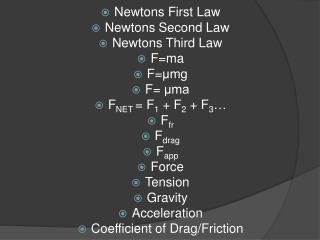DownloadDownload PresentationNewtons First Law Newtons Second Law Newtons Third Law F=ma F= μmg F= μma F NET = F 1 + F 2 + F 3 … F fr F drag F

# Newtons First Law Newtons Second Law Newtons Third Law F=ma F= μmg F= μma F NET = F 1 + F 2 + F 3 … F fr F drag F

Télécharger la présentation## Newtons First Law Newtons Second Law Newtons Third Law F=ma F= μmg F= μma F NET = F 1 + F 2 + F 3 … F fr F drag F

- - - - - - - - - - - - - - - - - - - - - - - - - - - E N D - - - - - - - - - - - - - - - - - - - - - - - - - - -
##### Presentation Transcript

1. Newtons First Law Newtons Second Law NewtonsThird Law F=ma F=μmg F= μma FNET = F1 + F2 + F3… Ffr Fdrag Fapp Force Tension Gravity Acceleration Coefficient of Drag/Friction
2. ### Gravitation & Kepler’s Laws

3. Kepler’s Laws Kepler’s First Law: Planets orbit in Ellipses Kepler’s Second Law: Planets travel distances that cover equal amounts of area in the ellipse per month.
4. Kepler’s Laws Kepler’s Third Law: The ratio of two planets periods (squared) is equal to the ratios of distances of the two planets to the sun (cubed). (TA/TB)2 = (rA/rB)3 T = period (time for one trip around the sun) r = distance to sun
5. Newton’s Law of Universal Gravitation Gravitational Force is a field force that is based on an amount of mass two objects have which make them attract to each other. FGravitation = G (m1m2/r2) G = 6.67E-11 Nm2/kg2 M1 or M2 = mass of objects one and two R = distance between two centers of the two masses
6. Orbits Period of a Planet Orbiting the Sun or Satellite orbiting the earth: T = 2π√(r3/Gm) G = 6.67E-11 Nm2/kg2 M= mass of the sun for a planet orbiting the sun or mass of earth for a satellite orbiting the earth R = orbiting radius